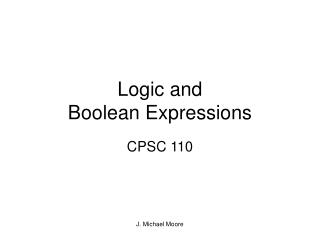DownloadDownload PresentationLogic and Boolean Expressions

# Logic and Boolean Expressions

Download Presentation## Logic and Boolean Expressions

- - - - - - - - - - - - - - - - - - - - - - - - - - - E N D - - - - - - - - - - - - - - - - - - - - - - - - - - -
##### Presentation Transcript

1. Logic and Boolean Expressions CPSC 110 J. Michael Moore

2. Decision-Making With Multiple Expressions • Format: if (Boolean-expression) logical-operator (Boolean-expression) then body; • Example: if (x > 0) AND (y > 0) then writeln ('X is positive, Y is positive'); J. Michael Moore

3. Decision-Making With Multiple Expressions (2) • Built-in logical operators in Pascal • _____ • _____ • _____ • _____ • (NAND and NOR can be constructed by combining _____ with _____ & _____ with _____) J. Michael Moore

4. Compound Boolean Expressions With “OR” Operator • Format: if (Boolean-expression) OR (Boolean-expression) then body; • Example: if (gpa > 3.7) OR (yearsJobExperience > 5) then writeln(‘You are hired’); J. Michael Moore

5. Compound Boolean Expressions With “AND” Operator • Format: if (Boolean-expression) AND (Boolean-expression) then body; • Example: if (yearsOnJob <= 2) AND (isGoofOff = True) then writeln(‘You are fired’); J. Michael Moore

6. Compound Boolean Expressions With “XOR” Operator • Format: if (Boolean expression) XOR (Boolean expression) then body; • Example: if (takesFirstJob = true) XOR (takesSecondJob = true) then isEmployed := true; OR if (takesFirstJob) XOR (takesSecondJob) then isEmployed := true; J. Michael Moore

7. Compound Boolean Expressions With “NOT” Operator • Format: if NOT (Boolean expression) then body; • Examples: if NOT (x AND y) then writeln(‘NAND’); if NOT (x OR y) then writeln(‘NOR’); J. Michael Moore

8. Order Of The Operations Order/Priority Operator • ___ • ___ ___ ___ ___ ___ • ___ ___ ___ • ___ ___ ___ ___ ___ ___ J. Michael Moore

9. AND has ___________ so the ‘0’ and ‘y’ become operands for this operation Why Bracket Boolean Expressions • Compound Boolean expressions • e.g., if x > 0 AND y > 0 then J. Michael Moore

10. Range Checking • What about doing (x < y < z) to check if y is in the proper range? • Use _______________________ J. Michael Moore

11. Quick Summary: Using Multiple Expressions • Use multiple expressions when multiple Boolean expressions must be asked and the result of each expression may have an effect on the other expressions: • AND: • All Boolean expressions must evaluate to true before the entire expression is true. • If any expression is false then whole expression evaluates to false • OR: • If any Boolean expression evaluates to true then the entire expression evaluates to true. • All Boolean expressions must evaluate to false before the entire expression is false. J. Michael Moore